## solve for x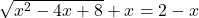please show all workings​

Question

solve for xin progress 0
2 months 2021-07-30T08:24:52+00:00 2 Answers 3 views 0

1. Step-by-step explanation:

Hey there!

Given;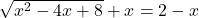Take “X” in right side.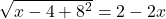Squaring on both sides;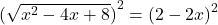Simplify;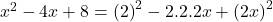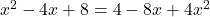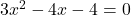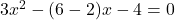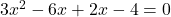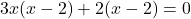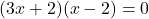Either;

3x+2 = 0

x= -2/3

Or;

x-2 = 0

x= 2

Check:

Keeping X= -2/3,

√(x²-4x+8 ) +X = 2-x

√{(-2/3)²-4*-2/3+8}+(-2/3) = 2+2/3

8/3 = 8/3 (True)

Now; Keeping X= 2

√{(2)²-4*2+8}+2 = 2-2

8 ≠0 (False)

Therefore, the value of X is -2/3.

Hope it helps!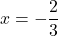Step-by-step explanation:

We want to solve the equation: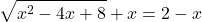We can isolate the square root. Subtract x from both sides: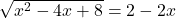And square both sides: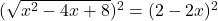Expand: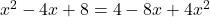Isolate the equation: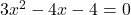Factor: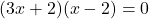Zero Product Property: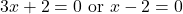Solve for each case. Hence: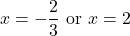Now, we need to check for extraneous solutions. To do so, we can substitute each value back into the original equation and check whether or not the resulting statement is true.

Testing x = -2/3: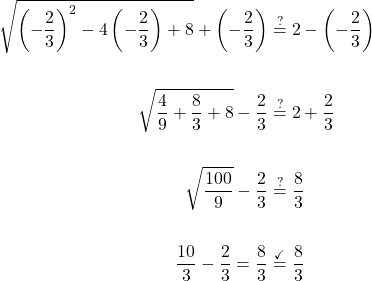Since the resulting statement is true, x = -2/3 is indeed a solution.

Testing x = 2: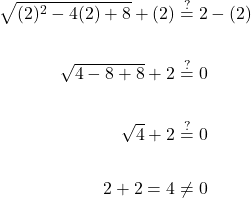Since the resulting statement is not true, x = 2 is not a solution.

Therefore, our only solution to the equation is x = -2/3.